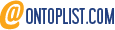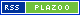9jaswitch

9jaswitch is an informational blog encouraging you to learn something new and expand your knowledge base.

Home » Properties of a Triangle and How to Apply its Formulas

# Properties of a Triangle and How to Apply its Formulas

What are the basic properties of a triangle, the Congruence, its types, and how to apply its formulas in equations and solutions

## What is a Triangle

A triangle is a polygon that has three closed angles. According to the classification of internal angles, there are three types of triangles – Equilateral, Isosceles, and Scalene.

Additionally, there are six types of triangles in total – Isosceles, Scalene, Equilateral, Oblique, Acute, and Right. A triangle can be classified by the length of its sides.

## What are the Properties of a Triangle

The six basic properties of a triangle

• Vertex
• Base
• Altitude
• Median
• Area
• Perimeter

### 1 Vertex:

Vertices are the corners of triangles. Every triangle has three vertices

### 2. Altitude

Triangles have a perpendicular altitude from their base to their opposite vertex.

• Since there are three possible bases, there are also three possible altitudes.
• The three altitudes intersect at a single point, called the orthocenter of the triangle.

### 3. Base:

In a triangle, the base can be any of the three sides, but it is generally the side nearest center.

• You can choose any side to serve as the base.
• Commonly used to calculate the area of the triangle.
• As a rule, the base of an isosceles triangle is considered to be unequal.

## 4. Area

A triangle’s area is calculated by multiplying its base by its height. The formula below can be used to calculate the area of a triangle.

• R: Radius of the circumscribed circle
• b: length of the base
• c: the other sides
• h: is the length of the corresponding altitude
• r: is the radius of the inscribed circle
• P: is the perimeter

### 5. Median

An equilateral triangle’s median is the line connecting its vertex to the midpoint of its opposite side

## What are the Properties of the Medians in a Triangle

• In a triangle, three medians intersect at the centroid
• Divided into two smaller triangles, each median has the same area as the other.
• The triangle has its center of gravity at its centroid (the point where they meet).
• Triangles are divided into six equal-sized triangles by the three medians.
• In each median, two-thirds of its length is located between the vertex and the centroid, and one-third lies between the centroid and the opposite side’s midpoint.
• Triangles always intersect at the same point no matter what shape they take.
• a, b and c are the sides of the triangle

### 6. Perimeter

Equilateral triangles have the largest area for a given perimeter. For a given area, equilateral triangles have the smallest perimeter.

## Properties of a Triangle by Angles

### 1. Scalene triangle

All the sides and angles of the scalene triangle differ from each other

### 2. Equilateral triangle

what is an equilateral triangle? Equilateral triangles are also regular polygons having 60-degree angles.

• At any point P in an equilateral triangle, the sum of the perpendiculars to the three sides is equal to the altitude.
• Equilateral triangles have the largest area for any given perimeter.

### 3. Isosceles triangle

An isosceles triangle also has two angles of the same measure; namely, the angles opposite to the two sides of the same length.

To find the base given the leg and altitude, use the formula

To find the leg length given the base and altitude, use the formula

To find the altitude given the base and leg (Where: L = the length of a
leg; A = the altitude, B = the length of the base)

### 4. Right triangle

In a right triangle, the hypotenuse is equidistant from the three polygonal vertices. A right triangle can also be isosceles if both sides of the right angle are the same length

• The hypotenuse of a right triangle is always longer than the other two sides, thus it can never be equilateral.

## How to Find the Area of a Right Triangle

Pythagoras Theorem defines the relationship between the three sides of a right triangle:

where is the c length of the hypotenuse and a, b are the lengths of the other two sides

### 5. Obtuse/Oblique Angle Triangle

Obtuse angle triangles have one angle that measures more than 90°.

Take Away: You may also be interested in reading the following advanced level articles on triangles

• Triangle congruence theorems and Similar Triangles
• Properties of the incenter of a triangle and circumcenter of a triangle### Amos

This site uses Akismet to reduce spam. Learn how your comment data is processed.error: Content is protected !!